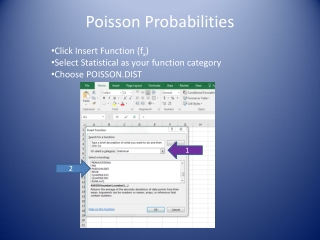DownloadDownload PresentationPoisson Probabilities

# Poisson Probabilities

Télécharger la présentation## Poisson Probabilities

- - - - - - - - - - - - - - - - - - - - - - - - - - - E N D - - - - - - - - - - - - - - - - - - - - - - - - - - -
##### Presentation Transcript

1. Poisson Probabilities Click Insert Function (fx) Select Statistical as your function category Choose POISSON.DIST 1 2

2. Poisson Probabilities You must enter X = Number of occurrences of interest Mean = Expected number of occurrences Cumulative = True if you would like a cumulative probability (P(x<X)) = False if you would like an individual point probability

3. Poisson Probabilities Example One: During weekday mid-morning hours, phone calls arrive at a motel chain's 800 number reservation center at the rate of 60 per hour. The probability of a call arriving is the same during any two periods of equal length during these hours. The arrival or nonarrival of a call in any time period is independent of the arrival or nonarrival of a call in any other time period of equal length during these hours. What is the probability of at least five calls in a 10-minute period during weekday mid-morning hours? Here we have a Poisson process with a mean of 10 (calls per 10-minute period). We need P(x > 5). Using the complement, P(x > 5) = 1 – P(x < 4). Use Excel to get P(x < 4). X=4 Mean = 10 Cumulative = True P(x < 4) = .0293, so the answer to our question is 1-.0293 = .9707

4. Poisson Probabilities Example Two: During weekday mid-morning hours, phone calls arrive at a motel chain's 800 number reservation center at the rate of 60 per hour. The probability of a call arriving is the same during any two periods of equal length during these hours. The arrival or nonarrival of a call in any time period is independent of the arrival or nonarrival of a call in any other time period of equal length during these hours. What is the probability of exactly five calls in a 10-minute period during weekday mid-morning hours? Here we have a Poisson process with a mean of 10 (calls per 10-minute period). We need P(x = 5). So enter X=5 Mean = 10 Cumulative = False The answer to our question is .0378